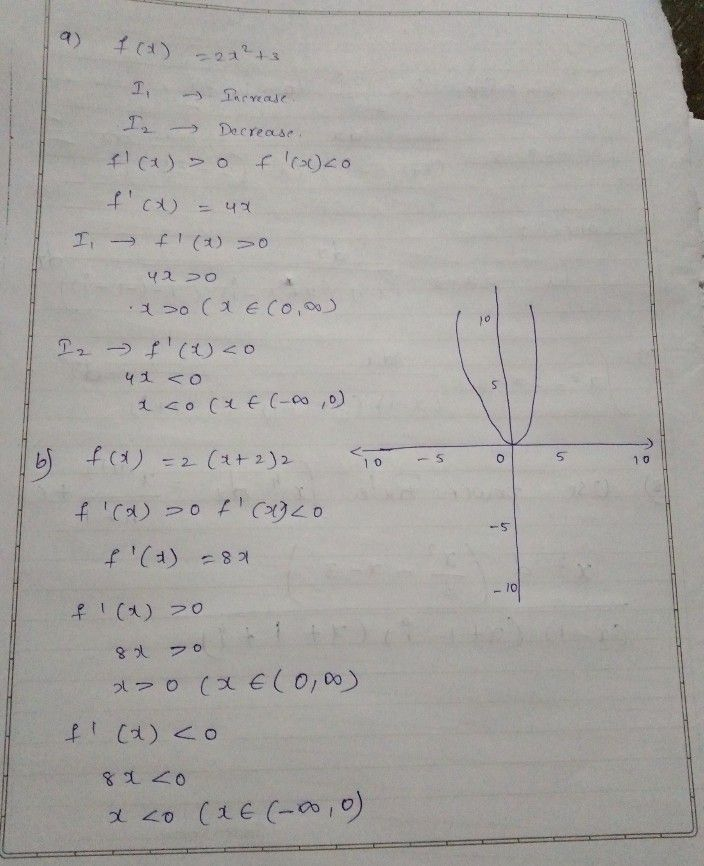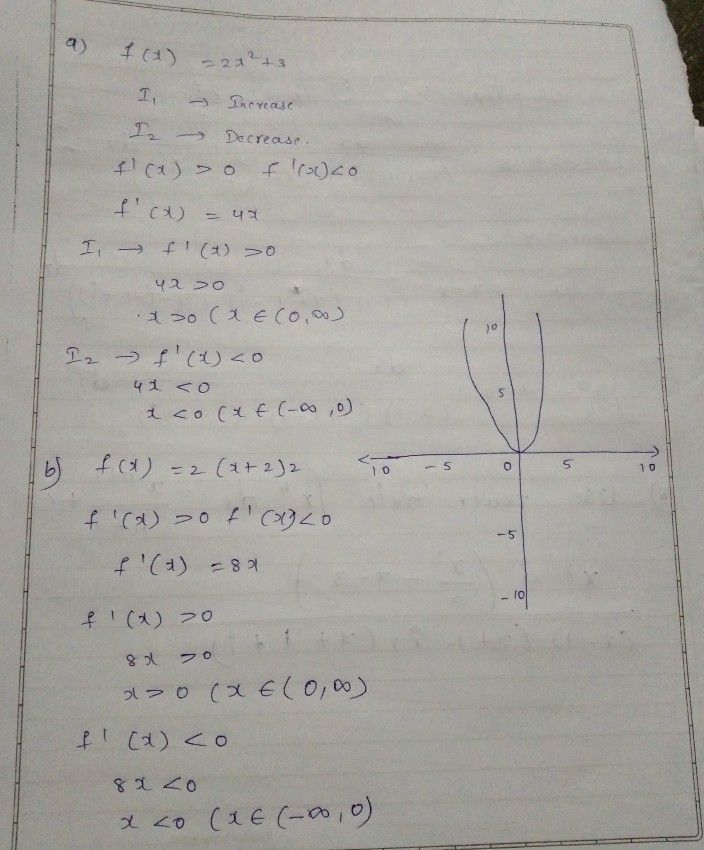Symbol
Problem$B$ The graph of $f\left(x\right)=2x2$ is shown below. Based on this graph, sketch the graphs of the following quadratic functions in the same coordinate system. $\square .$ $f\left(x\right)=2x^{2+3}$ b. $f\left(x\right)=2\left(x+2\right)2$ $10$ $5$ $-10$ $-5$ $0$ $5$ $10$ $-5$ $-10$
Other
Search count: 112
Question content